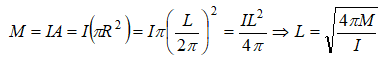A straight wire carrying current i is turned into a circular loop. If the magnitude of magnetic moment associated with it in M.K.S. unit is M, the length of wire will be

(a) $4\mathrm{\pi iM}$                          (b) $\sqrt{\frac{4\mathrm{\pi M}}{i}}$

(c) $\sqrt{\frac{4\mathrm{\pi i}}{M}}$                        (d) $\frac{M\mathrm{\pi }}{4i}$

Concept Videos :-

#2 | Magnetic Field & Magnetic Field Lines

Concept Questions :-

Magnetic field and field lines

Magnetic moment of circular loop carrying currentDifficulty Level:

• 8%
• 60%
• 23%
• 11%